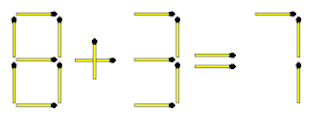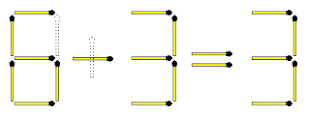## Drop down Tab menu for Site

### FIGURE OUT PUZZLES - matchstick puzzles (04)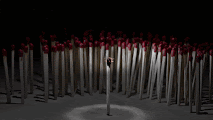QUESTION 1
The above equation is not true. Move only 1 matchstick to make it true.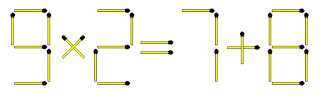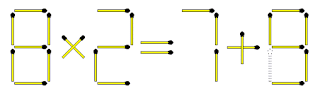QUESTION 2
The equation 7/9 = 3 is not true. Move 3 matchsticks to make it true.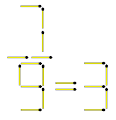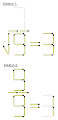QUESTION 3
Move only 1 matchstick to create 4.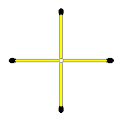Solution 1 is the better solution.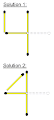QUESTION 4
The above equation is not true. Move only 3 matchsticks to make it true.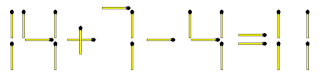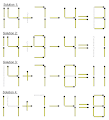QUESTION 5
The above statement is true. Move 2 matchsticks so that it is still true.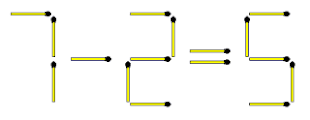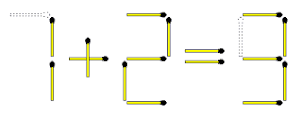QUESTION 6
In each row the letter to the left of the arrow converts to the structure to the right. What should the structure of the "?" look like in the last row?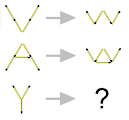The matchsticks on the lower half of each letter are flipped vertically.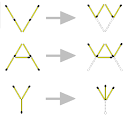QUESTION 7
5 plus 5 is not equal to 9, but move 4 matchsticks to make the equation true.QUESTION 8
10 plus 10 is not equal to 5, but move only 3 sticks to make the equation true. Your answer must still be in Roman numerals.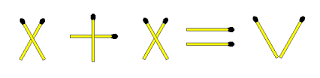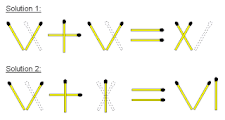QUESTION 9
There are 4 cubes in the figure above. Confirm that you agree.
Move only 1 matchstick to reduce the number of cubes to 3.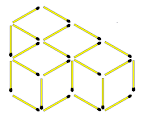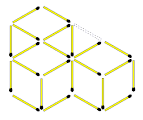QUESTION 10
A school of fish swim in an Easterly direction. By moving only 3 matchsticks they must swim in a Westerly direction. (The matchstick heads must be ignored in this puzzle)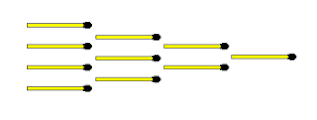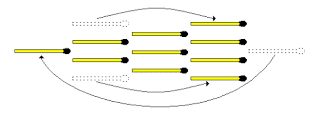QUESTION 11
Rearrange the six matchsticks to make NOTHING.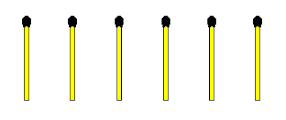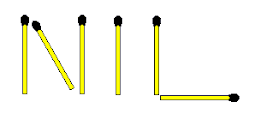QUESTION 12
The equation above is not true. You may not take away a matchstick, but simply MOVE 1 matchstick to make it true.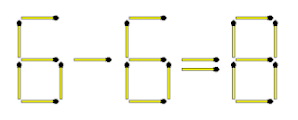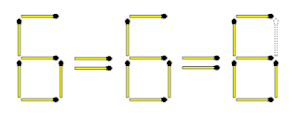QUESTION 13
There are 5 squares in the figure above. Move only 2 matchsticks so that there are:
(1) no squares left AND
(2) 4 areas equal in size and shape.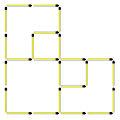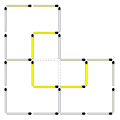QUESTION 14
In each row the structure to the left of the arrow converts to the structure to the right. What should the structure of the "?" look like in the last row?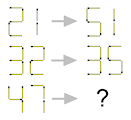Nobody said these are numbers! Look closely at the matchstick heads. The structures to the right of the arrows are all flipped vertically.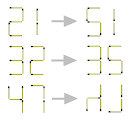QUESTION 15
The equation is not true, but by moving only 1 matchstick (from the "+" sign) you get 7-1=6 which makes the equation true.
This is easy, but can you MOVE 1 and REMOVE 1 to come up with a different answer?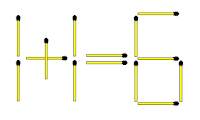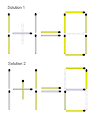QUESTION 16
The equation above is not true, but REMOVE 2 matchsticks to make it true.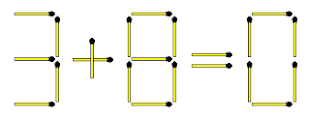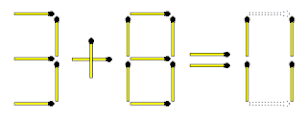QUESTION 17
Remove 5 matchsticks from the figure above to make it 2.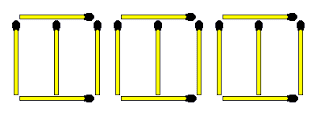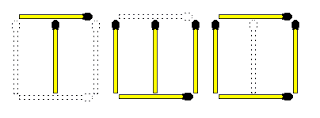QUESTION 18
There are 5 squares in the figure above. Move only 2 matchsticks to create 4 squares. All the squares don't have to be equal in size.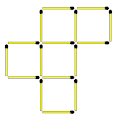There are also other solutions.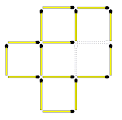QUESTION 19
The time on a digital watch is 30 minutes past 4. Move only 2 matchsticks to show this time.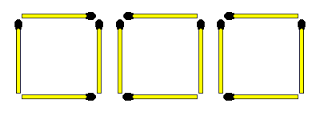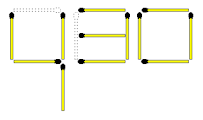QUESTION 20
Move 2 matchsticks to make the equation true.